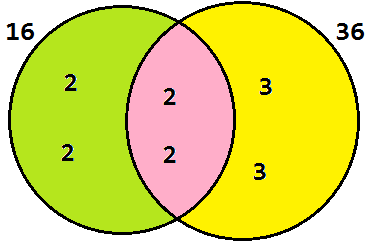# HOW TO FIND HCF AND LCM OF TWO COMPOSITE NUMBERS?

HOW TO FIND HCF AND LCM OF TWO COMPOSITE NUMBERS?
How to find Highest Common Factor and Least Common Multiple of two composite numbers?
For example, we want to find HCF and LCM of 16 and 36, from this factor tree animation below

we will getChoose the smallest number for each set to find the HCF

so, HCF of 16 and 36 is 2^2=4.
And choose the highest number for each set to find the LCM

so, LCM of 16 and 36 is 2^4x3^2=144.

Or we can use the Venn Diagram belowBased on Venn Diagram above, we put 2, 2, 2, and 2 as the factors of 16, and put 2, 2, 3 and 3 as the factors of 36.

And the shaded pink region as common factors of 16 and 36
so, HCF of 16 and 36 is 2.2=4 (multiply only the common factors)
and LCM of 16 and 36 is 2.2.2.2.3.3=144 (multiply all factors togethers)

Lets learn maths. :)